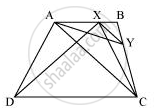# ABCD is a trapezium with AB || DC. A line parallel to AC intersects AB at X and BC at Y. Prove that ar (ADX) = ar (ACY). - Mathematics

ABCD is a trapezium with AB || DC. A line parallel to AC intersects AB at X and BC at Y. Prove that ar (ADX) = ar (ACY).

[Hint: Join CX.]

#### SolutionIt can be observed that ΔADX and ΔACX lie on the same base AX and are between the same parallels AB and DC.

∴ Area (ΔADX) = Area (ΔACX) ... (1)

ΔACY and ΔACX lie on the same base AC and are between the same parallels AC and XY.

∴ Area (ΔACY) = Area (ACX) ... (2)

From equations (1) and (2), we obtain

Concept: Corollary: Triangles on the same base and between the same parallels are equal in area.
Is there an error in this question or solution?

#### APPEARS IN

NCERT Class 9 Maths
Chapter 9 Areas of Parallelograms and Triangles
Exercise 9.3 | Q 13 | Page 164